### Home > PC > Chapter 1 > Lesson 1.3.1 > Problem1-98

1-98.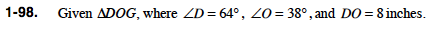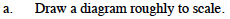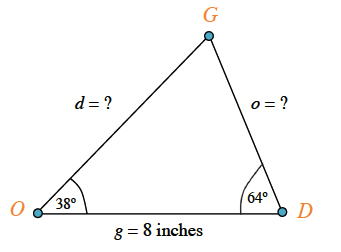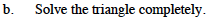Make a chart like the one below. It helps you quickly 'see' what needs to be figured out as well as helping you quickly find a strategy.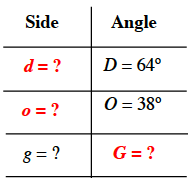Determine the measure of angle G first. Then use the Law of Sines to calculate the missing side lengths.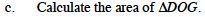Draw a height from vertex G ⊥ to side OD.

Using your calculation for side o and the sine function for angle D, find the height. Then use the area formula for a triangle.